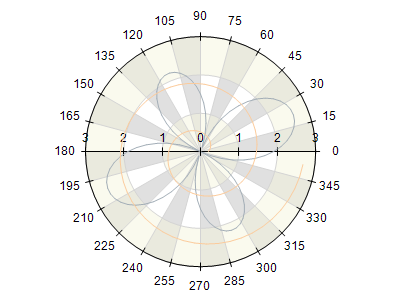﻿ Angular Scale Configurator
Nevron .NET Vision Documentation
Angular Scale Configurator

Angular scales are primarily used in polar charts in order to scale angles in the polar model space. In order to scale angles a scale has to be configured in a way that addresses the specifics of the angle values in general as well as the specifics of the angle unit used to describe a given angle. There are two major considerations when you scale angles and they arise from the fact that angles are cyclic (for example the angle 370 degrees is equal to 10 degrees):

- The scale must apply cyclic transformation for logical to scale units (for example the projected value of 370 degrees and 10 degrees must be the same).
- When using automatic labels the scale must choose a step which is a divisor of the angle cycle length. This improves the readability of the scale as the scale is symmetrically decorated when looking at it from right to left and left to right.

The following picture shows a polar chart where these two considerations are addressed:Note that the step in this case is chosen to be 15, which is a divisor of the range [0, 360]. The scale also does not decorate the value 360 as it already decorated with 0.

Angle Unit

The NAngularScaleConfigurator has a property called AngleUnit, allowing you to specify the unit of measurement for angles. By default it is set to Degrees, but you can also choose from Grads and Radians. The following code snippet changes the angle unit of a polar angle scale:

C#
Copy Code
NPolarAxis axis = (NPolarAxis)polarChart.Axis(StandardAxis.PolarAngle);
NAngularScaleConfigurator angularScale = new NAngularScaleConfigurator();
axis.ScaleConfigurator = angularScale;
Visual Basic
Copy Code
Dim axis As NPolarAxis = CType(polarChart.Axis(StandardAxis.PolarAngle), NPolarAxis)
Dim angularScale As New NAngularScaleConfigurator
axis.ScaleConfigurator = angularScale
Cycle Length

When the control decorates the scale with an angle step, from a readability standpoint it is better to choose a step which is a divisor of the range [0, 90], instead of [0, 360] (in degrees). This is because the user intuitively expects to have the 0, 90, 180 and 270 degrees annotated. By default the angular scale is configured to choose a divisor in the range [0, 90], but you can change that using the cycle legnth property. The following code will change the cycle length for decoration to 0, 180:

C#
Copy Code
NPolarAxis axis = (NPolarAxis)polarChart.Axis(StandardAxis.PolarAngle);
NAngularScaleConfigurator angularScale = new NAngularScaleConfigurator();
axis.ScaleConfigurator = angularScale;
Visual Basic
Copy Code
Dim axis As NPolarAxis = CType(polarChart.Axis(StandardAxis.PolarAngle), NPolarAxis)
Dim angularScale As New NAngularScaleConfigurator
angularScale.CycleLength = New NAngle(90, NAngleUnit.Degree)
axis.ScaleConfigurator = angularScale

The effect of this code is that now the step 60 is also allowed (60 is not a divisor of 90, but is a divisor of 180).